# HDU6030 Happy Necklace[矩阵快速幂]

## Happy Necklace

HDU - 6030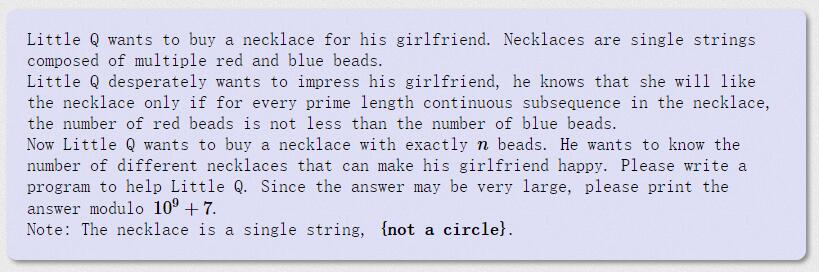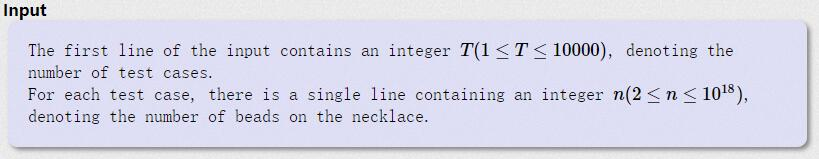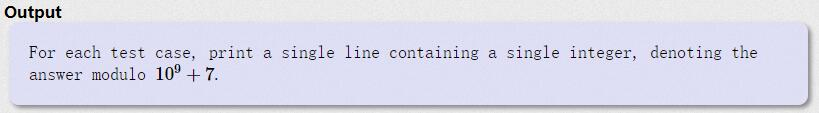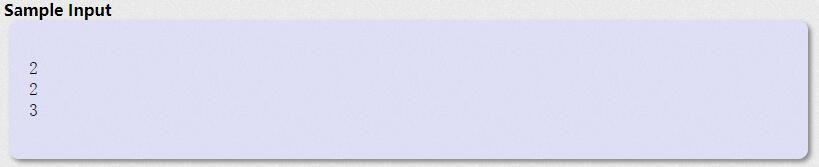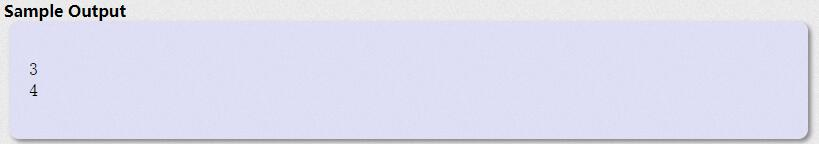① ox  的后面，只能接 o. 会构成 oxo

② xo 的后面，也只能接 o 会构成 xoo

③ oo 的后面，既可以接 o ，也可以接 x。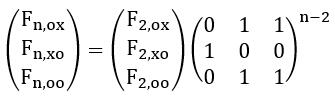(渣渣的人生第一次一血啊T_T[在女生专场里]  哇 刚看到  来补补博客纪念一下)

#pragma comment(linker, "/STACK:102400000,102400000")
#include<iostream>
#include<cstdio>
#include<cstring>
#include<cmath>
#include<string>
#include<algorithm>
#include<queue>
#include<stack>
#include<set>
#include<map>
#include<vector>
using namespace std;
typedef long long ll;
const int mod=1e9+7;
const int N=5;
ll ans[N][N],a[N][N],tmp[N][N];
void initial()
{
memset(ans,0,sizeof(ans));
memset(a,0,sizeof(a));
for (int i=0 ; i<3 ; ++i)
ans[i][i]=1;
a=a=a=a=1;
}
void Pow(ll a[][N],ll b[][N],int n)
{
memset(tmp,0,sizeof(tmp));
for (int i=0 ; i<n ; ++i)
for (int j=0 ; j<n ; ++j)
if (a[i][j])
for (int k=0 ; k<n ; ++k)
tmp[i][k]=(tmp[i][k]+a[i][j]*b[j][k]%mod)%mod;
for (int i=0 ; i<n ; ++i)
for (int j=0 ; j<n ; ++j)
a[i][j]=tmp[i][j];
}
void Pow(ll a[][N],ll m)
{
while (m)
{
if (m&1)
Pow(ans,a,3);
Pow(a,a,3);
m>>=1;
}
}
int main()
{
int T;
scanf("%d",&T);
while (T--)
{
ll n;
scanf("%lld",&n);
initial();
ll res=0;
Pow(a,n-2);
for (int i=0 ; i<3 ; ++i)
for (int j=0 ; j<3 ; ++j)
res=(res+ans[i][j])%mod;
printf("%lld\n",res);
}
return 0;
}